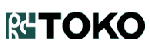电子元器件数据表 IC PDF查询English中文版 品牌 我要上传
 型号： 开始 精确 包含 结束 描述：
SN74ALS29861  S25FL128SAGBHIG10  SZ2530  SOD1E8  SZ1012  S25FL128S  S80C188EB20  SZ1018  SOD1F1  SZ1033
TK71726SCL/26 低压差稳压器 (LOW DROPOUT VOLTAGE REGULATOR).型号：   TK71726SCL/26
PDF文件： 下载PDF文件   鼠标右键选目标另存为

LOW DROPOUT VOLTAGE REGULATOR

Logo：PDF原版 中文翻译版100%TK717xxS

n

mF

n
n
n
n
n
n
n
n
n
n
n
n

OUT
³
1.8 V)

= 103毫伏的
100 mA时）

Q
= 72微安的我
OUT
= 0 mA时）

mA

n
n
n
n
n
n

72低静态电流
mA

50 mA负载。待机电流典型值为100 pA的。该

μF的;
a

TK717xxS
VIN
GND
VOUT

TK717xxS是具有内置低压差线性稳压器

TTL或CMOS逻辑电平。器件处于导通状态

mV
RMS
。内部PNP旁路晶体管

01 S

TK717

。S L
VIN
VOUT

-
+
TAPE / REEL CODE

15 = 1.5 V
16 = 1.6 V
17 = 1.7 V
18 = 1.8 V
19 = 1.9 V
20 = 2.0 V
21 = 2.1 V
22 = 2.2 V
23 = 2.3 V
24 = 2.4 V
25 = 2.5 V
26 = 2.6 V
27 = 2.7 V
28 = 2.8 V
29 = 2.9 V
30 = 3.0 V
31 = 3.1 V
32 = 3.2 V
33 = 3.3 V
34 = 3.4 V
35 = 3.5 V
36 = 3.6 V
37 = 3.7 V
38 = 3.8 V
39 = 3.9 V
40 = 4.0 V
41 = 4.1 V
42 =
43 =
44 =
45 =
46 =
47 =
48 =
49 =
50 =
4.2 V
4.3 V
4.4 V
4.5 V
4.6 V
4.7 V
4.8 V
4.9 V
5.0 V
TAPE / REEL CODE
L：带左

C: -30 ~ 80°C

S： SOT23-5

AUTO

GND

2001年10月TOKO公司

 首页 - - 友情链接 Copyright© 2001 - 2014 ICPDF All Rights Reserved ICPDF.COM粤公网安备 44030402000629号粤ICP备13051289号-7